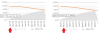## Recommended Posts

Hi, I'm triyng to plot a chart with percentage values, but when the value is below 1% it's displayed incorrectly on mouse over.

See the xml below, the second (fev/12) value is 1,64% and is displayed correctly in the chart, but the first value (jan/12), that should be 0,89%, is displayed incorrectly (it's displayed 0,01, without the '%' symbol after the number)

Is there a way to fix this?

I attached a screenshot.

```<chart decimals='2' forceDecimals='1' decimalSeparator=',' inDecimalSeparator=',' numberScaleValue='.01' numberScaleUnit='%'>

<categories>
<category label='jan/12' />
<category label='fev/12' />
<category label='mar/12' />
<category label='abr/12' />
...

<dataset seriesname='CDI YTD' renderas='Area' color='d9d9d9' alpha='70' showPlotBorder='0'>
<set value=',00887327' />
<set value=',01635646' />
<set value=',02459936' />
<set value=',03180139' />
...

</chart>
```

Tks!